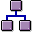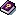Alternative Valuation Run: Process FlowProcess Flow

1. Execute aSelection of materials and activities per plant.
2. If you have chosen a starting period (under General data -> Period data), that is before the alternative valuation run periods, execute a cumulation. The data is cumulated over several periods, this means that the data from the categories Receipts, Consumption and Other Receipts and Consumption is added together for all of the periods to be cumulated.
3. The system calculates the sequence that the materials will be costed in.
4. Execute thesingle level material price determination (based on the cumulative data).
5. If you have selected the option 'Actual prices for period' as the price (under General data ® Settings), complete price determination.
6. The system determines the (cumulative) prices, based on the CO versions you created.
7. Executemultilevel material price determination, (based on the cumulative data).
8. If you have set the indicators posting run and revaluate consumption (under General data ® settings), execute the revaluation of consumption.
9. The system determines the delta postings.

In this process step, the system determines the differences between the ending inventory price in the alternative valuation run and the ending inventory price in the final period of the periodic costing run. The delta postings are then statistically updated.

1. Make the closing entry.

The delta values determined in the previous step are posted to delta balance sheet accounts in Financial Accounting.